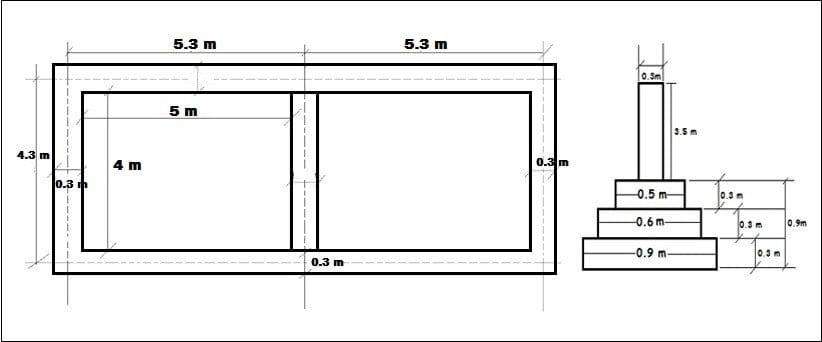# Center Line Method for Calculating the Quantities of Building Materials

Estimating is a predictive approach of calculating various quantities and corresponding expenditure for the particular project. Estimation provides an idea about cost of work, time required for completion and hence its feasibility can be determined. Estimation is mostly done using the following methods:

• Long wall short wall method.
• Centre line method.
• Crossing method.

The centerline method can be effectively used in Load bearing buildings. That means in which walls are constructed as load bearing structures. Since the foundation is also constructed by brick work only and there are no separate coloumns in the structure, this method can be useful for quickly calculating the amount of building material (mainly bricks) used for house construction.

This method cannot be easily used to calculate the material requirement in framed structures (i.e., where coloumn, beam and foundations are used to building a house).

Here, we will be discussing about Centre line method along with a sample.

## What is Center Line Method

Centre line method is adopted for walls of similar cross sections. In centre line method, total centre line length of all the walls is first measured, provided walls are of same type, long and short having same cross section and same type of footings. After the calculation of centre line length, it is multiplied with the breadth and depth correspondingly to find the total quantity. Using centre line method provides an edge with higher work pace; however, extra attention must be paid at junctions, meeting points of partition or cross walls, etc.

• Centre line method proves to be very simple for rectangular, circular, polygonal buildings which have no cross walls.
• In case of buildings with cross walls or partition walls, proper consideration must be given at every junction to calculate correct quantity.
• In case of buildings with one partition wall or cross wall having two junctions, for earthwork in foundation trench and foundation concrete, deduction of one breadth of trench or concrete from total centre length. For each junction half breadth of the respective item is to be deducted from the total centre length.
• For footings, deduction of one breadth from the total centre length is to be done for two junctions.
• In case of number of footings, the length of first footing is determined by applying half breadth as deduction per junction from the total centre line length and for subsequent footing, simply offset for every junction to the length of previous footing.
• At every stage deduction of half breadth of the main wall at that particular level shall have to be made per junction i.e. overall one breadth for two junctions from the total centre line length, and this net centre length after deduction shall be multiplied by the respective breadth and depth to get total quantities.
• If two walls come from opposite directions and they meet at the same point, there will be two junctions.
• For buildings having different type of walls, each set of walls have to be considered separately. All the outer walls or main walls shall be considered first together and then all inter or cross walls must be considered. There is no provision of deduction for main walls or outer walls. However, deduction of half breadth of main wall, for each junction must be made for inter or cross walls.
• At corners of building where two walls meet, there is no provision for addition or deduction.

## A Sample Calculation of 2 room Building• Total centre line length = 2 x ( Length of main wall 1 + Length of main wall 2 ) + 3 x ( Length of partition wall )

Total centre line length = 2 x (5.3 + 5.3) + 3 x 4.3 = 34.1 m

• Earthwork in excavation = Total centre line length x Breadth x Depth.

= 34.1 x 0.9 x (3 x 0.3)

= 34.1 x 0.9 x 0.9

= 27.621 m3.

• Concrete in foundation = Total centre line length x Breadth x Depth.

= 34.1 x 0.9 x 0.3

= 9.207 m3.

• Brickwork in foundation for first footing = Total centre line length x Breadth x Depth.

= 34.1 x 0.6 x 0.3

= 6.138 m3.

• Brickwork in foundation for second footing = Total centre line length x Breadth x Depth.

= 34.1 x 0.5 x 0.3

= 5.115 m3.

• Brickwork in plinth = Total centre line length x Breadth x Depth.

= 34.1 x 0.3 x 0.6

= 6.138 m3.

• Brickwork in superstructure = Total centre line length x Breadth x Depth.

= 34.1 x 0.3 x 3.5

= 35.805 m3.

After the calculation of these basic quantities, we can easily estimate the quantities for various items to be used like quantity of plaster, quantity of paint, quantity of damp proof course, etc, by using total centre line length and respective dimensions.

– Tushar Meena